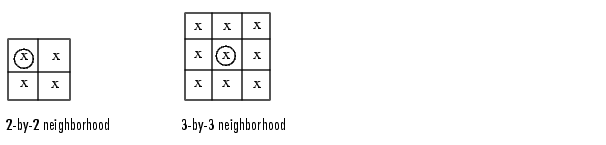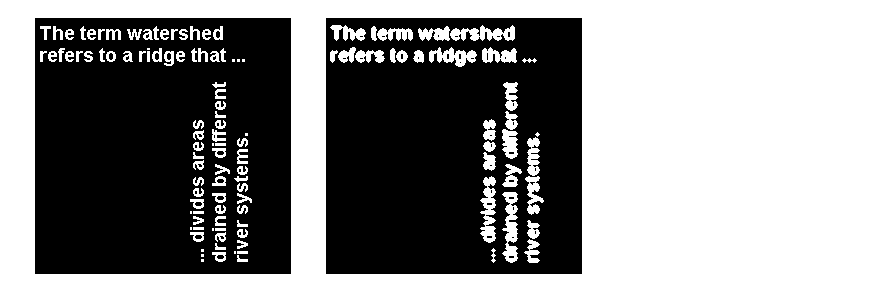Image Processing Toolbox User's GuideLookup Table Operations

Certain binary image operations can be implemented most easily through lookup tables. A lookup table is a column vector in which each element represents the value to return for one possible combination of pixels in a neighborhood.

You can use the `makelut` function to create lookup tables for various operations. `makelut` creates lookup tables for 2-by-2 and 3-by-3 neighborhoods. This figure illustrates these types of neighborhoods. Each neighborhood pixel is indicated by an x, and the center pixel is the one with a circle.For a 2-by-2 neighborhood, there are 16 possible permutations of the pixels in the neighborhood. Therefore, the lookup table for this operation is a 16-element vector. For a 3-by-3 neighborhood, there are 512 permutations, so the lookup table is a 512-element vector.

Once you create a lookup table, you can use it to perform the desired operation by using the `applylut` function.

The example below illustrates using lookup table operations to modify an image containing text. You begin by writing a function that returns 1 if three or more pixels in the 3-by-3 neighborhood are 1; otherwise, it returns `0`. You then call `makelut`, passing in this function as the first argument, and using the second argument to specify a 3-by-3 lookup table.

• ```f = inline('sum(x(:)) >= 3');
lut = makelut(f,3);
```

`lut` is returned as a 512-element vector of 1's and 0's. Each value is the output from the function for one of the 512 possible permutations.

You then perform the operation using `applylut`.

• ```BW1 = imread('text.png');
BW2 = applylut(BW1,lut);
imshow(BW1)
figure, imshow(BW2)```

Image Before and After Applying Lookup Table Operation

For information about how `applylut` maps pixel combinations in the image to entries in the lookup table, see the reference page for `applylut`.

 Note    You cannot use `makelut` and `applylut` for neighborhoods of sizes other than 2-by-2 or 3-by-3. These functions support only 2-by-2 and 3-by-3 neighborhoods, because lookup tables are not practical for neighborhoods larger than 3-by-3. For example, a lookup table for a 4-by-4 neighborhood would have 65,536 entries.Finding the Euler Number of a Binary Image Analyzing and Enhancing Images